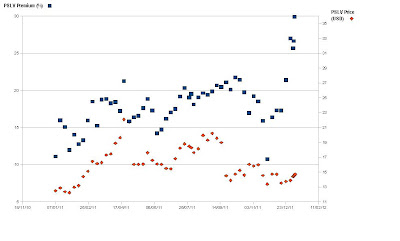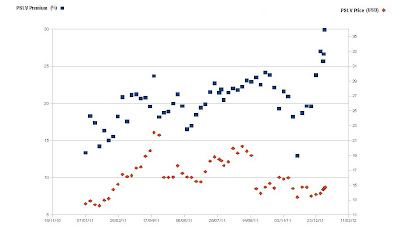## zaterdag 7 januari 2012

Today I fabricated a nice chart of the PSLV price (Right Y-axis) and the PSLV premium (Left Y-axis) from the beginning of 2011 till today.

I calculated this as follows:
I first calculated a standardization coefficient based on the premium, price of PSLV and SLV of 30 December 2011. The standardization coefficient was 2.55 (30 December 2011) and 2.5 (5 January 2012). If I had the historical premiums I could make an average standardization coefficient. The problem is that I don't have the data for historical premiums of PSLV...

So we'll just go with this premium here to calculate Stand. coefficient (30 December 2011):

SLV price
Stand. coefficient = --------------------------------------------------------
(PSLV price / (1+ PSLV premium*0,01))

= 26.94 / (13.43/(1+ 27*0.01)) = 2.55

Based on this coefficient I calculated the PSLV premium to spot price with this formula:

PSLV Premium = ((PSLV price * Coefficient ) / SLV price) -1

Then I plotted this PSLV price /PSLV premium chart in function of time for 2011.PSLV premium (%) and Price (USD) in function of time (coefficient 2,5)PSLV premium (%) and Price (USD) in function of time (coefficient 2,55)

As you can see there is a correlation between the PSLV price and the PSLV premium. When the premium goes up (blue squares), then the PSLV price follows a few days later (orange diamonds).

So the premium of PSLV is a good indication to know if the spot silver price will go up or down. When the PSLV premium goes up, then the PSLV price (and also the spot silver price) will go up a few days later and vice versa. Also note that the premium trend is going up, which is a bullish sign for silver.

Conclusion: Always watch what the premium is doing. The latest days the premium shot up to 30%, which means in the future we will see a huge price spike. Let's see if my predictions are correct. :-)